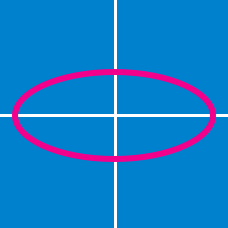Geometry

# Conics - Circle Standard Equation

What is the equation of the circle passing through the points $(0,0)$ and $(6,12)$ with center on the line $y=x+3?$

What is the equation of the circle which passes through the two points $(-3,0)$ and $(7,4)$ and is centered at a point on the $y$-axis?

If $(-2, -6)$ and $(8, 8)$ are two endpoints of a diameter of a circle, what is the equation of the circle?

What is the equation of the circle tangent to the $x$-axis with center $(5,-1)$?

If the circle $x^2+y^2-8x+2y +1=0$

has center $(a,b)$ and radius $r,$ what is the value of $a+b+r ?$

×